Latest Banking jobs   »   Revision Test Basic Quantitative Aptitude Quiz...

# Revision Test Basic Quantitative Aptitude Quiz for All Banking Exams- 17th May

Q1. If profit obtained on selling an article is equal to % above the C.P. at which the article sold then find C.P (in Rs)?
(a) None of these
(b) 200
(c) 300
(d) Cannot be determined
(e) 100

Q2. Ram and Shyam together can do a work in 16 days. Shyam takes twice time as Ram takes to do the same work. In how many days Shyam alone can complete that work.
(a) 16 days
(b) 32 days
(c) 24 days
(d) 40 days
(e) 48 daysL1Difficulty 2
QTags Time And Work
QCreator Deepak

Q3. P, Q and R together can do a work in 27 days. They together work for 17 days and after that R left the work and the remaining work is done by P and Q together in 16 ⅔ days. Work done by P in 4 days is equal to work done by R in 3 days. Then, Find time taken by P alone to do this work ?
(a) 90 days
(b) 80 days
(c) 100 days
(d) 95 days
(e) 105 days

Q4. Rajat invested Rs 6,000 at rate of interest 20% per annum. The interest was compounded yearly for the first year and in the second year it was compounded half yearly. What will be the total interest earned at the end of the second year?
(a) Rs 2924
(b) Rs 2784
(c) Rs 2824
(d) Rs 2624
(e) Rs 2712

Q5. A shopkeeper sold an article at a profit of 1/8th of its SP to a person. If shopkeeper sold it at 25% more than previous selling price it will have Rs 68 more as profit. Find its selling price.
(a) Rs. 280
(b) Rs. 272
(c) Rs. 290
(d) Rs. 300
(e) Rs. 264

Q6. Ratio of marked price to cost price of an item is 5 : 3. Discount percent given on selling that item which is equal to the profit percent earned on that item. Find marked price is what percent of selling price of that item ?
(a) 120%
(b) 125%
(c) 133 ⅓%
(d) 140%
(e) 150%

Q7. The ratio of numerical value of rate of interest and time period at a sum invested is 5 : 2 and men get 22.5% of invested sum as interest. Find at the same rate how much interest man will get on the sum of Rs. 1600 for two year ?
(a) 240 Rs.
(b) 180 Rs.
(c) 160 Rs.
(d) 120 Rs.
(e) 224 Rs.

Q8. A, B and C invested in a ratio of 7 : 8 : 5 in a business. They got an annual profit of Rs. 136800. If A and C withdrew their amount at the end of 3 months and 7 months respectively then find the difference between A and C’s share of profit?
(a) Rs. 12,600
(b) Rs. 11,500
(c) Rs. 13,500
(d) Rs. 10,500
(e) Rs. 13,000

Q9. Cost price of three type of laptop are in the ratio of 3 : 5 : 2 respectively. If the profit percent on three laptops are 25%, 10% and 20% respectively then find the overall profit percentage is?
(a) 19%
(b) 22%
(c) 15%
(d) 16.5%
(e) 25%

Q10. Sumit’s investment is 1.5 times the investment of Sahil and ratio between period of their investment is 2 : 1. If share of Sumit in total profit is Rs.7550 more than the share of Sahil in total profit, then find the total profit.
(a) Rs.7550
(b) Rs.15100
(c) Rs.14100
(d) Rs.13500
(e) Rs.15000

Q11. 5 years hence Vikas will 4 years older than Rahul. Ratio between present age of Rahul and Amit is 10 : 11. If Amit is 2 years younger than Vikas then find the sum of present ages of all three.
(a) 64
(b) 60
(c) 56
(d) 66
(e) 65

Q12. Sita has two sons named Luv and Harry. The ratio of Sita and Harry’s present age is 5 : 1 and ratio between Sita and Luv’s age 2 year ago was 3 : 1. If Luv is 6 years elder than Harry, Find Sita’s age when Luv was born?
(a) 35 yrs
(b) 28 yrs
(c) 25 yrs
(d) 20 yrs
(e) 22 yrs

Q13. A 40 ℓ mixture contain water and milk in the ratio 3 : 2. How much milk must be added to the mixture such that amount of water and milk become equal in the mixture.
(a) 16 ℓ
(b) 24 ℓ
(c) 8 ℓ
(d) 4 ℓ
(e) 12 ℓ

Q14. A shopkeeper has two quality rice. Rice A is sold at Rs. 40 per kg and Rice B is sold at Rs. 25 per kg. He mixed both the rice together and sold the mixture at Rs. 36 per kg. Find the ratio of quantity of rice A to the total mixture ?
(a) 11 : 4
(b) 4 : 11
(c) 4 : 15
(d) 11 : 15
(e) None of these

Q15. A certain sum of money becomes thrice of itself in 4 years on SI. Find the time period in which it becomes 8 times of itself ?
(a) 7 yrs
(b) 14 yrs
(c) 6 yrs
(d) 12 yrs
(e) 28 yrs

Practice More Questions of Quantitative Aptitude for Competitive Exams:

Solutions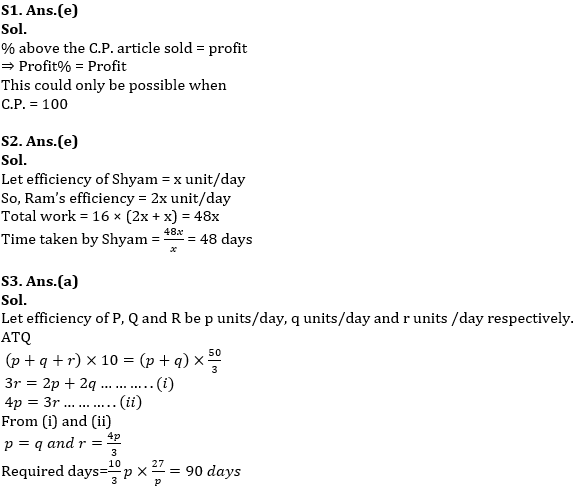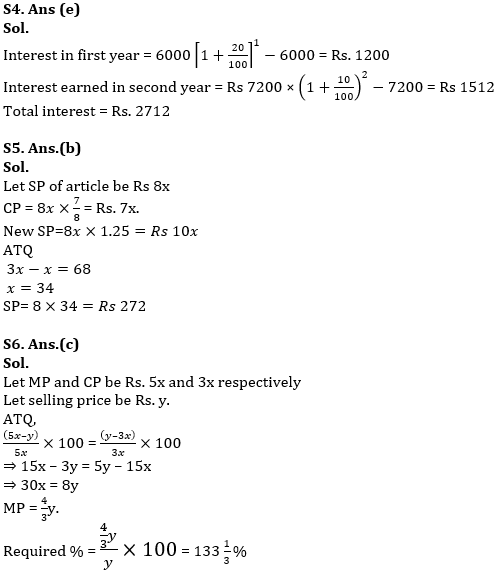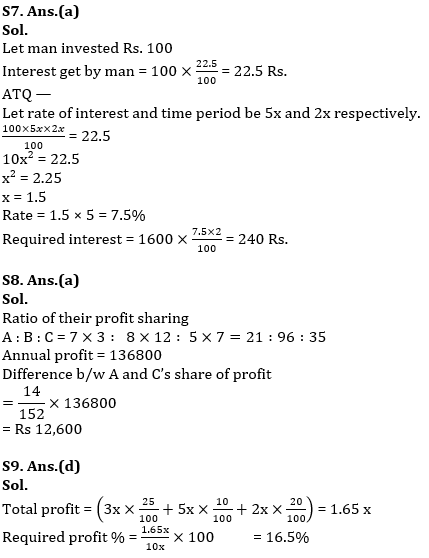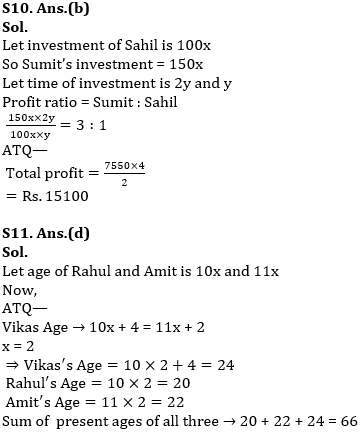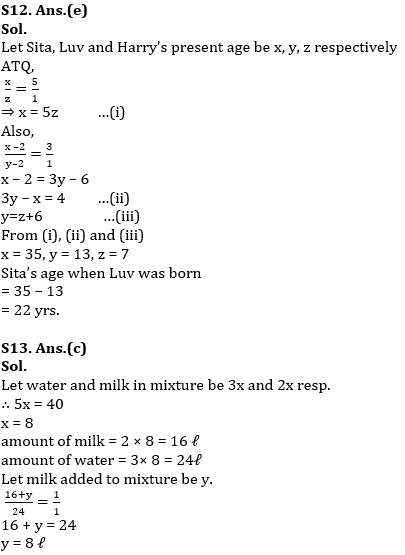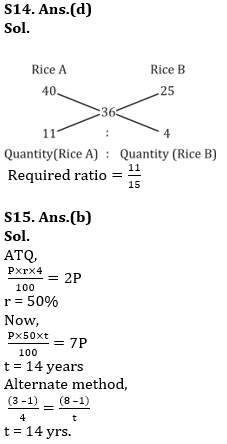#### Congratulations!Incorrect details? Fill the form again here

•Important Current Affairs Quiz for Bank ...
•Important Current Affairs Quiz for Bank ...
•Reasoning Ability Quiz For IBPS RRB PO C...
•Quantitative Aptitude Quiz For IBPS RRB ...
•Important Current Affairs Quiz for Bank ...
•Reasoning Ability Quiz For IBPS Clerk Pr...